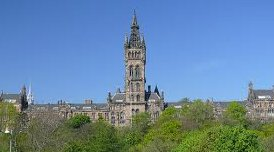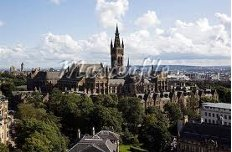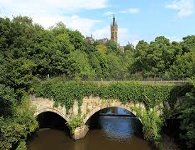Four different views of Glasgow University Tower

# Conference Two-Graphs

The files
as their titles suggest, contain all conference two-graphs on at most 50 vertices that are known to me. Some of these two-graphs are in fact regular two-graphs and are duplicated here. Each two-graph is identified with its greatest descendant, where the ordering involved is the natural one obtained by expressing as a binary integer the concatenation of the rows of the upper triangular part of the adjacency matrix. I also list the order of its automorphism group, and the orbits under the action of this group. In addition, I include the numbers of strongly regular graphs that are obtained either as descendants of the conference two-graph or, where appropriate, in its switching class. The strongly regular graphs are identified by their parameters which comprise the number of vertices and the eigenvalues of their Seidel Spectrum. This is simply the spectrum of its (-,+) adjacency matrix. The notation [x,y] means that there are x graphs with automorphism group of order y.

Perhaps the updated files concerning conference two-graphs on 38, 42 and 46 vertices need some explanation. In  the method used to determine all regular two-graphs on 36 vertices was adapted to generate 191 conference twographs on 38 vertices, with similar results for 42, while Mathon  found 17 self-complementary strongly regular graphs on 45 vertices that give rise to the same number of self-complementary regular two-graphs on 46 vertices, and the additional work  increased the known number of conference two-graphs on 50 vertices to 54 (6 self-complementary and 24 complementary pairs).

 Brendan McKay & E Spence, The Classification of Regular Two-graphs on 36 and 38 vertices, Australas. J. Combin.. 24 (2001), 293-300.

 R, Mathon, On self-complementary strongly regular graphs, Discrete Math. 69 (1988), 263-281.

 R, Mathon, On self-complementary strongly regular graphs, Discrete Math. 69 (1988), 263-281.

 E. Spence, Unpublished computer result, 1995.